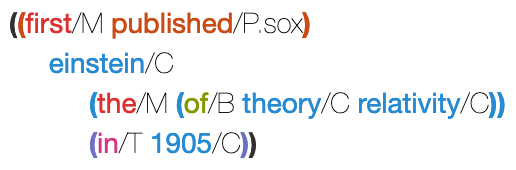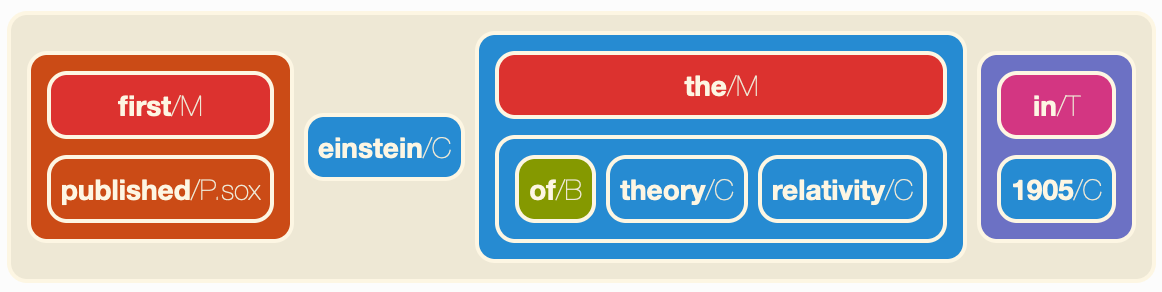# Notebooks and visualizations

The `graphbrain.notebook` package contains functions that are specifically meant for use with Jupyter notebooks. Currently it provides three hyperedge visualizations, meant to be used inside notebooks.

The function `show()` produces a indented visualization with syntax highlighting according to hyperedge type:

```from graphbrain.notebook import *
show('((first/M published/P.sox) einstein/C (the/M (of/B theory/C relativity/C)) (in/T 1905/C))')
```

The optional argument `style` specifies layout style. The two possible options are “indented” (the default) and “line”.The function `blocks()` produces a block diagram, also using colors to distinguish hyperedge types:

```blocks('((first/M published/P.sox) einstein/C (the/M (of/B theory/C relativity/C)) (in/T 1905/C))')
```The function `vblocks()` also produces a block diagram similar to `blocks()`, which is more vertically spread and might thus be preferable for complex hyperedges:

```from graphbrain import hedge
edge = hedge("""(reports/Pd.sx wikipedia/C (that/T
(and/J
((first/M published/Pd.sox) einstein/Cp.s (the/M (of/B theory/C relativity/C)) (in/T 1905/C))
(revolutionized/P.o physics/C))))""")
vblocks(edge)
```Both `blocks()` and `vblocks()` accept the optional arguments “subtypes” (default: False), “argroles” (default: True) and “namespaces” (default: False). These are meant to control the level of detail at which atoms are represented.